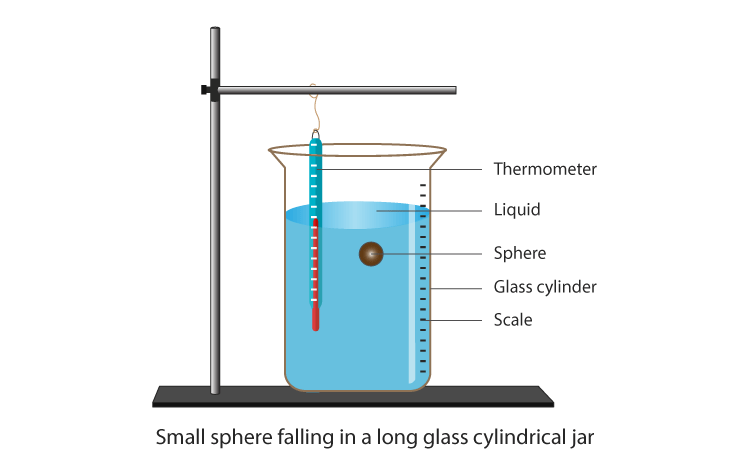# To determine the coefficient of viscosity of a given viscous liquid by measuring the terminal velocity of a given spherical body

Coefficient of viscosity is used to calculate the viscosity of the liquid. Below is an experiment on how to determine the coefficient of viscosity of a given viscous liquid by measuring the terminal velocity of a given spherical body.

## Aim

To determine the coefficient of viscosity of a given viscous liquid by measuring terminal velocity of a given spherical body.

## Materials Required

1. A half metre high
3. Transparent viscous liquid
4. One steel ball
5. Screw gauge
6. Stop clock/watch
7. Thermometer
8. Clamp with stand

## Theory

### What is terminal velocity?

Terminal velocity is defined as the highest velocity that is attained by an object as it falls through a fluid. When the sum of drag force and buoyancy are equal to the force of gravity, terminal velocity occurs.

### What is terminal velocity formula?

Following is terminal velocity formula:

 $$\begin{array}{l}v=\frac{2}{9}\frac{r^{2}(\rho -\sigma )}{\eta }\end{array}$$

Where,

• v is the terminal velocity
• r is the radius of the spherical body
• g is the acceleration due to gravity
• ρ is the density of the spherical body
• σ is the density of the liquid
• η is the coefficient of viscosity

## Diagram## Procedure

1. Clean the glass jar and fill it with transparent viscous liquid.
2. The vertical scale along the height of the jar must be clearly visible to note the least count.
3. Check the tight spring of the stopwatch and also record the least count and zero error.
4. Record the least count and zero error of the screw gauge.
5. Determine the radius of the ball.
6. Gently drop the ball in the liquid. Initially, the ball falls with an accelerated velocity until it reaches one-third height of the liquid. Then it falls with uniform terminal velocity.
7. As the ball reaches some convenient division, start the stopwatch to note its fall.
8. As the ball reaches the convenient lowest division, stop the stopwatch.
9. Record the time taken by the ball.
10. Repeat steps 6 to 9 twice for more readings.
11. Record the temperature of the liquid.
12. Record the observations.

## Observations

Least count of vertical scale = 1 mm

Least count of stop clock/watch = …….. s

Zero error of stop clock/watch = ……. s

Pitch of screw gauge (p) = 1mm

No.of divisions on the circular scale = 100

Least count of screw gauge (LC) = 1/100 = 0.01 mm

Zero error of screw gauge (e) = …… mm

Zero correction of screw gauge (c) = (-e) = ….. mm

The diameter of the spherical ball

• Along one direction, D1 = ….. mm
• In the perpendicular direction, D2 = …….. mm

Terminal velocity of spherical ball

Distance fallen, S = …… cm

Time taken,

t1 = …….. s

t2 = …….. s

t3 = …….. s

## Calculations

Mean diameter,

$$\begin{array}{l}D=\frac{D_{1}+D_{2}}{2}\;mm\end{array}$$

$$\begin{array}{l}r=\frac{D}{2}\,mm\,=\,……\,cm\end{array}$$

Meantime,

$$\begin{array}{l}t=\frac{t_{1}+t_{2}+t_{3}}{3}\,=\,…..\,s\end{array}$$

Mean terminal velocity,

$$\begin{array}{l}v=\frac{S}{t}\,=\,….\,cm.s^{-1}\end{array}$$

From formula,

$$\begin{array}{l}\eta =\frac{2r^{2}(\rho -\sigma )g}{9v}=\,……\,C.G.S.units\end{array}$$

## Result

The coefficient of viscosity of the liquid at a temperature (T℃) = ……. C.G.S.units

## Precautions

1. To watch the motion the liquid used must be transparent
2. The perfectly spherical ball should be used
3. Velocity should be noted only when it is constant

## Sources Of Error

1. The density of the liquid used must be non-uniform
2. The ball used might not be perfectly spherical
3. Velocity noted might not be constant

## Viva Questions

Q1. Why the liquid must be transparent?

Ans: In order to see the motion of the ball, the liquid must be transparent.

Q2. How does the viscosity of liquid and gases change?

Ans: As the temperature increases, the viscosity of the liquid decreases and the viscosity of gas increase with the increase in temperature. When the pressure increases, the viscosity of liquid increases and the viscosity of the gas does not get affected.

Q3. Why does hot water move faster than cold water?

Ans: Viscosity of hot water is smaller than that of cold water. Therefore, hot water moves faster than cold water.

Q4. What is the dimensional formula of dynamic viscosity?

Ans: Following is the dimensional formula of dynamic viscosity:

 [M1L-1T-1]

Q5. What is the CGS unit of dynamic viscosity?

Ans: Poise is the CGS unit of dynamic viscosity.